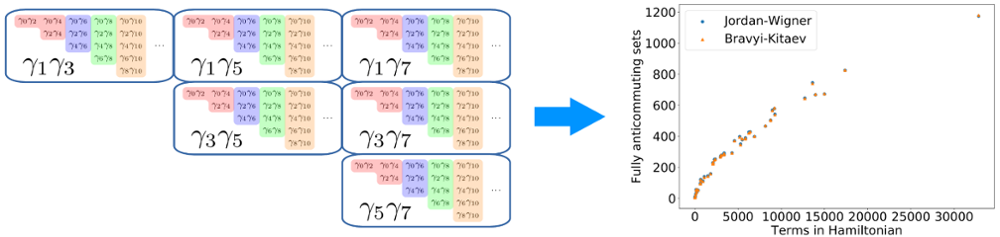# Reducing the measurement complexity of variational quantum algorithms

June 16, 2020 - Andrew ZhaoAndrew Zhao and Akimasa Miyake, with collaborators from Tufts University and Caltech, have published a paper in Physical Review A in which they propose a technique to reduce the number of circuit repetitions required to implement variational quantum algorithms. Such algorithms are designed for the hardware limitations of present-day quantum computers. Instead of performing one long, sophisticated quantum circuit, variational algorithms repeatedly run and measure the outputs of shorter, more feasible circuits, and then use classical optimization techniques to arrive at a solution. Typically, one uses these algorithms to calculate physical quantities, such as the energy of a chemical system.

However, the number of variational circuit repetitions required can be overwhelming, especially when simulating chemistry. To address this issue, the paper introduces a method to group together compatible measurements which may be performed simultaneously, in exchange for slightly longer circuits. To demonstrate the practical effectiveness of this technique, the authors present numerical calculations in the context of simulating various chemical molecules. They observe a roughly tenfold reduction in the number of required measurements, and they furthermore confirm this effectiveness in general with analytical proofs.